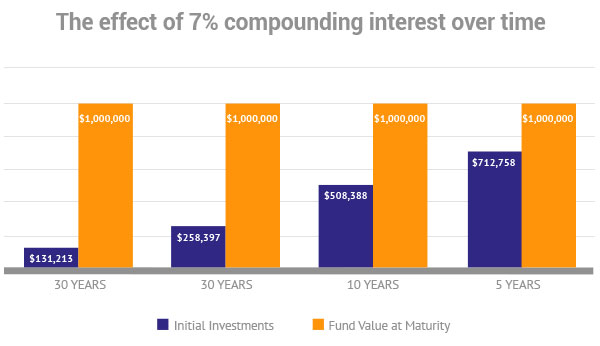# Compound interest equals free money

April 30, 2015 / Knowledge Centre

Interest which is calculated not just on the initial principal but also the accumulated interest of prior periods. Another common explanation is “Interest over Interest”So why is compounding interest so important? Because effectively it equates to free money! The above example illustrates very well the need to start saving early and to save long-term in order to get the best effect.

For example, if you want to achieve \$1.000.000 at 7% compounding interest and have 30 years to reach this figure you will need to start with \$131.213, or approximately 13% of the final amount.

Should you delay the start with 10 years your personal investment required has grown to \$258.397, or almost 30% of the final amount. That delay effectively cost you \$12.718 per year which equates to \$1.060 per month!!!

The morale of the above story is start saving now as it is so much more rewarding when you have time on your side. The longer you wait the harder it will get.

The formula to calculate compound interest is: P = C(1+ r/n)(n*t)

Where:
P = future value
C = initial deposit
r = interest rate (expressed as a fraction: eg. 0.06 for 6%)
n = # of times per year interest is compounded
t = number of years invested

To make life easier we have done the calculations for you and produced the table below: